## 本文应用场景

#### 表 1. 部分患者心脏检查样本数据## 逻辑回归简介

#### 图 1. 逻辑回归## 建立逻辑回归模型的步骤

1. 构造假设函数（即 H

1. 构造损失函数（即 J 函数）

1. 通过某种方法使得损失函数最小，并求得此时的参数θ

### 构造假设函数

#### 图 2. Sigmoid 函数曲线Sigmoid 函数将输入 z 转化为一个 0 到 1 之间的数，正好对应为概率的值，g(z)越趋近于 1，表示结果为 1 的概率越大，且概率值就等于 g(z)，反之，当 g(z)越趋近于 0，表示结果为 0 的概率越大，且概率值等于 1- g(z)。有了 Sigmoid 函数，我们可以构造预测函数了，在上一篇文章 《机器学习代码实战：使用线性回归检测水泥质量》 中，我们介绍了有多个自变量的线性回归的公式可表示为：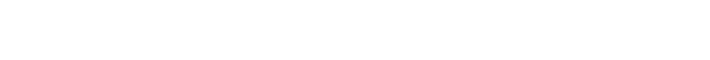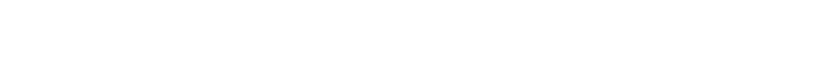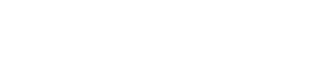### 构造损失函数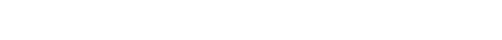### 梯度下降求θ1. 每次迭代只对参数有比较小的更新，要经过多次迭代才能得到最终理想的参数。

1. 如果步长太大，可能会在最低点附近徘徊，所以训练模型的时候要注意调整步长。

1. 这个方法让你走到的不一定是整个曲面的最低点，可能只是局部最小，所以往往要考虑起点。

### 向量化计算1.求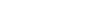2.求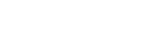3.求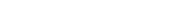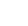表示矩阵 x 的转置矩阵）

## 模型的代码实现

#### 清单 1. 定义矩阵运算函数

```// 矩阵乘积: 左矩阵 * 右矩阵
public static float[][] times(float[][] left, float[][] right)
// 常数 a 与矩阵乘积: a * 矩阵
public static float[][] times(float a, float[][] matrix)
// 矩阵相加: 左矩阵 + 乘数 * 右矩阵
public static float[][] add(float[][] left, float multiplier, float[][] right)
// 转置矩阵
public static float[][] transpose(float[][] matrix)
// 计算数量积
public static float dotProduct(float[] left, float[] right)
// 计算行列式的值
public static float detValue(float[][] det)
// 提取矩阵的余子式
public static float[][] cofactor(float[][] matrix, int row, int col, float[][] result)

### 准备数据

#### 图 3. 数据文件部分内容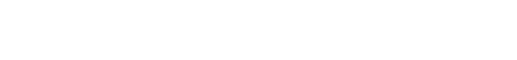#### 清单 2.数据处理过程

```// 数据文件
MatrixDataFile dataSource = new MatrixDataFile("/data/StatlogHeart.csv");
dataSource.featureScaling();
// 处理值数据
float[][] Y = dataSource.getY();
for(int i=0;i<Y.length;i++){
Y[i] = (Y[i]==1) ? 0:1 ;
}
// 训练与测试数据集
ArrayList<float[][]> trainData = dataSource.getFirst(200);
ArrayList<float[][]> testData  = dataSource.getLast(100);```

### 模型训练

#### 清单 3. 模型训练代码

```// 中间矩阵 a * xT
a_times_xT = Matrix.times(this.alpha, xT);
// 迭代
for(int times= 0; times<iterationTimes; times++){
// (1) 求 A = x * parameters;
Matrix.times(x, parametersT, A);
// (2) 求 E = g(A) - y
Calculate.sigmoid(A, g);

// (3) 求 parametersT := parametersT - a * xT * E
Matrix.times(a_times_xT, E, a_times_xT_times_E);
...
}```

### 训练监控

#### 清单 4.训练监控代码

```testData.get(1));LogicRegressionMonitor monitor = new LogicRegressionMonitor();
monitor.showResult(LR,100,1.2);```

## 模型训练实例

### 调超参数

#### 清单 5. 输入参数开始训练

```// 建立模型开始训练
LogicRegression LR = new LogicRegression();
LR.setXY(trainData.get(0),trainData.get(1));
LR.train(new float[]{1,1,1,1,1,  1,1,1,1,1,  1,1,1,  1}, 0.0015f, 2000);```

### 测试与监控

#### 图 4. 监控输出#### 图 5. 收敛曲线#### 图 6. 预测值与实际值对比曲线